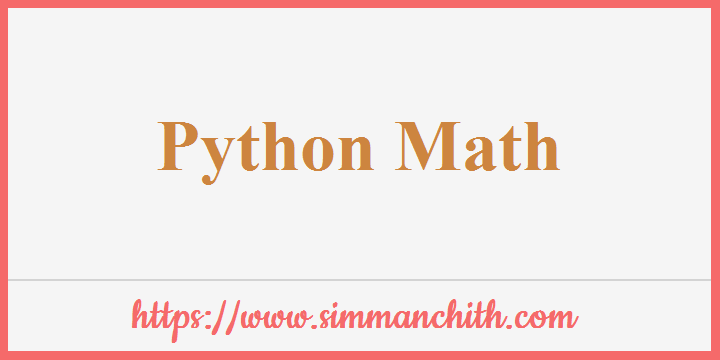# Python MathPython features a number of built-in `math` functions, including a large math module, which enables mathematical tasks to be carried out on numbers.

You can also search for these topics, python math function, Python Math problems, operate the python math, how to round the math in python, example for math in python, working of math function list using python, python combination of Math, python distance and expression in math function, python function parameter default value and type math is not defined, what are the package and programming language in python.

## Built-in Math Functions

Example 1 :- The functions `min()` and `max()` are used to find in an iterable the lowest or highest value :

``````x = min(5, 10, 25)
y = max(5, 10, 25)
print(x)
print(y)``````

Output :-

5
25

Example 2 :- The function `abs()` returns the absolute value (positive) of the provided number. It removes signs (positive (+) sign or negative (-) sign) from the given number.

``````x = abs(-7.25)
print(x)``````

Output :-

7.25

The function `pow(x,y)` returns the value x to the power y (xy) :

Example 3 :- Return the value of 4 to the power of 3 (same as 4 * 4 * 4):

``````x = pow(4, 3)
print(x)``````

Output :-

64

You can also search for these topics, python built in math function an error, math functions code in python math, math functions to verify the combination and format of python, built the function of math to define python, explain the python distance of built-in math functions, example for python built-in functions.

## The Math Module

Python additionally has an integrated `math` module which extends the mathematical list functions.

The `math` module has to be imported to use it :

``import math``

You can start with the methods and constants of the module when you have imported the `math` module.

Example 1 :- For example `math.sqrt()` returns a number's square root :

``````import math
x = math.sqrt(64)
print(x)``````

Output :-

8.0

Example 2 :- The function `math.ceil()` rounds a number up to its nearest integer, and the method `math.floor()` rounds down to its closest integer and returns the result :

``````#Import math library
import math
#Round a number upward to its nearest integer
x = math.ceil(1.4)
#Round a number downward to its nearest integer
y = math.floor(1.4)
print(x)
print(y)``````

Output :-

2
1

Example 3 :- The `math.pi` constant, PI value yields (3.14...) :

``````import math
x = math.pi
print(x)``````

Output :-

3.141592653589793

You can also search for these topics, python the math module source code, math module to install using python, python math module best practices, explain the code of python math module, check the division of module of math, example for python math module, working of math function in python module, python module math has no member, how to import the python module in math.